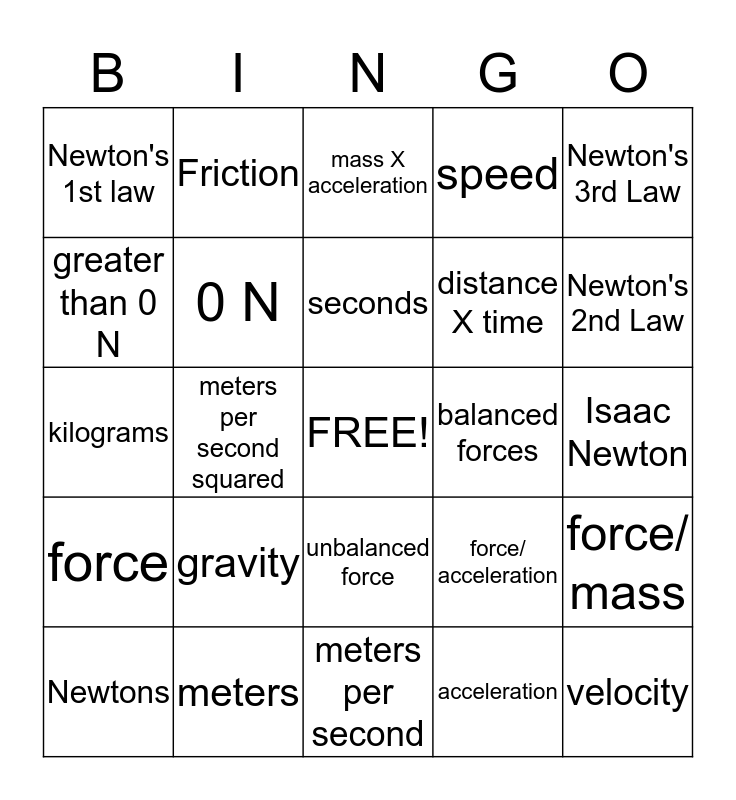# Physics BingoThis bingo card has a free space and 24 words: gravity, meters, seconds, Newtons, Newton's 3rd Law, Newton's 1st law, greater than 0 N, Friction, balanced forces, unbalanced force, distance X time, kilograms, force/ acceleration, force/ mass, 0 N, acceleration, Isaac Newton, mass X acceleration, Newton's 2nd Law, meters per second squared, velocity, force, speed and meters per second.

## Play Online